GATE  >  GATE Numerical Reasoning Aptitude (Analytical) Questions - 2

# GATE Numerical Reasoning Aptitude (Analytical) Questions - 2

Test Description

## 10 Questions MCQ Test General Aptitude for GATE | GATE Numerical Reasoning Aptitude (Analytical) Questions - 2

GATE Numerical Reasoning Aptitude (Analytical) Questions - 2 for GATE 2023 is part of General Aptitude for GATE preparation. The GATE Numerical Reasoning Aptitude (Analytical) Questions - 2 questions and answers have been prepared according to the GATE exam syllabus.The GATE Numerical Reasoning Aptitude (Analytical) Questions - 2 MCQs are made for GATE 2023 Exam. Find important definitions, questions, notes, meanings, examples, exercises, MCQs and online tests for GATE Numerical Reasoning Aptitude (Analytical) Questions - 2 below.
Solutions of GATE Numerical Reasoning Aptitude (Analytical) Questions - 2 questions in English are available as part of our General Aptitude for GATE for GATE & GATE Numerical Reasoning Aptitude (Analytical) Questions - 2 solutions in Hindi for General Aptitude for GATE course. Download more important topics, notes, lectures and mock test series for GATE Exam by signing up for free. Attempt GATE Numerical Reasoning Aptitude (Analytical) Questions - 2 | 10 questions in 15 minutes | Mock test for GATE preparation | Free important questions MCQ to study General Aptitude for GATE for GATE Exam | Download free PDF with solutions
 1 Crore+ students have signed up on EduRev. Have you?
GATE Numerical Reasoning Aptitude (Analytical) Questions - 2 - Question 1

### Fill in the missing value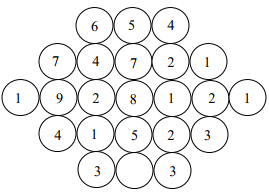Detailed Solution for GATE Numerical Reasoning Aptitude (Analytical) Questions - 2 - Question 1

Middle number is the average of the numbers on both sides.(Left and Right)
Average of 6 and 4 is 5
Average of (7 + 4) and (2 + 1) is 7
Average of (1 + 9 + 2) and (1 + 2 + 1) is 8
Average of (4 + 1) and (2 + 3) is 5
Therefore, Average of (3) and (3) is 3

The number appearing in the centre line is average of the sum of numbers appearing on left  and right of numbers.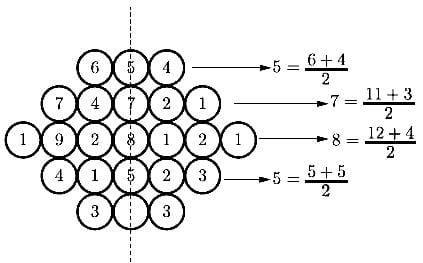Hence, the unknown number is given by 3 + 3 / 2 = 3

GATE Numerical Reasoning Aptitude (Analytical) Questions - 2 - Question 2

### Given (9 inches)1/2 = (0.25 yards) 1/2, which one of the following statements is TRUE?

Detailed Solution for GATE Numerical Reasoning Aptitude (Analytical) Questions - 2 - Question 2

Take square on both side, we get

9 inches = 0.25 yards

Why not option (A) ?

Let me give you an example

(3m)2=9m2

It means that if number gets squared then the units also get squared. Above is an example of a square having side 3m, the area of this square would be 9m2.

Similarly,

(9 m)½ = 3 m½

Hence, if I have

(9 inches)½ = (0.25 yards)½

If I take the square root, then we get

3 inches½ = 0.5 yards½

and not 3 inches = 0.5 yards

So option (A) is wrong

GATE Numerical Reasoning Aptitude (Analytical) Questions - 2 - Question 3

### M and N start from the same location. M travels 10 km East and then 10 km North-East. N travels 5 km South and then 4 km South-East. What is the shortest distance (in km) between M and N at the end of their travel?

Detailed Solution for GATE Numerical Reasoning Aptitude (Analytical) Questions - 2 - Question 3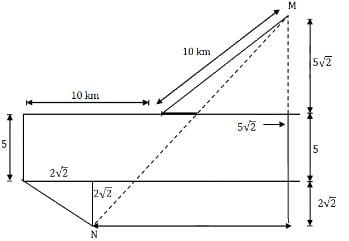So the adjoining figure for solution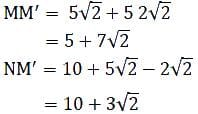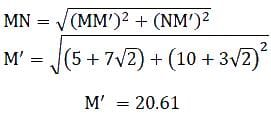GATE Numerical Reasoning Aptitude (Analytical) Questions - 2 - Question 4

The number that least fits this set: (324, 441, 97 and 64) is _____.

Detailed Solution for GATE Numerical Reasoning Aptitude (Analytical) Questions - 2 - Question 4

18= 324
21= 441
8= 64
X2 ! =  97
All the above are perfect squares but 97 is a prime number.

GATE Numerical Reasoning Aptitude (Analytical) Questions - 2 - Question 5

Find the area bounded by the lines 3x + 2y = 14, 2x - 3y = 5 in the first quadrant.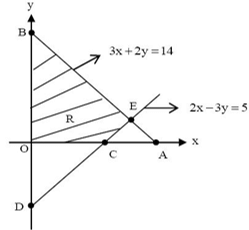Detailed Solution for GATE Numerical Reasoning Aptitude (Analytical) Questions - 2 - Question 5

The total area of the triangle which is bounded by the 2 given lines in the first quadrant

= 1/2 x 14/3 x 7

=  98 / 6

= 16.33 sq units

And area bounded with x axis and the lines in the 1st quadrant

= 1/2 x ( 4.67 - 2.5)

= 1.08 sq units

So area bounded with y axis  =  total area in 1st quadrant - area in 1st quadrant bounded by the lines and the   x axis

= 16.33 - 1.08

= 15.25 sq units

GATE Numerical Reasoning Aptitude (Analytical) Questions - 2 - Question 6

A straight line is fit to a data set (ln x, y). This line intercepts the abscissa at ln x = 0.1 and has a slope of −0.02. What is the value of y at x = 5 from the fit?

Detailed Solution for GATE Numerical Reasoning Aptitude (Analytical) Questions - 2 - Question 6

The equation of a line is
y = mx + c where m is the slope & c is the y-intercept
Now, In this question x is replaced with lnx
So, the equation of line becomes,
y = mlnx + c
or, y = -0.02lnx + c
We have given with abscissa which is essentially x-intercept. So, now we have to find ‘c’ the y-intercept.
for, y=0, lnx = 0.1 (given in the question)
Putting the value,
0 = -0.02 × 0.1 + c
or, c = 0.002
So, the equation of line becomes,
y = -0.02lnx + 0.002
putting x = 5 (asked in the question)
y = -0.002ln5 + 0.002 = -0.002 × 1.6 + 0.002 = -0.03
(ln5 = 1.6)

GATE Numerical Reasoning Aptitude (Analytical) Questions - 2 - Question 7

A cube of side 3 units is formed using a set of smaller cubes of side 1 unit. Find the proportion of the number of faces of the smaller cubes visible to those which are NOT visible.

Detailed Solution for GATE Numerical Reasoning Aptitude (Analytical) Questions - 2 - Question 7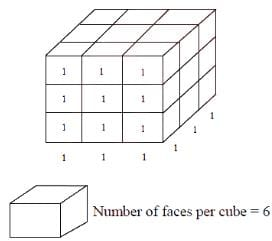Total number of cubes = 9 × 3 = 27
∴Total number of faces = 27 × 6 = 162
∴Total number of non-visible faces = 162 - 54 = 108

∴ Number of visible faces / Number of non visible faces = 54/108 = 1/2

GATE Numerical Reasoning Aptitude (Analytical) Questions - 2 - Question 8

Two and a quarter hours back, when seen in a mirror, the reflection of a wall clock without number markings seemed to show 1:30. What is the actual current time shown by the clock?

Detailed Solution for GATE Numerical Reasoning Aptitude (Analytical) Questions - 2 - Question 8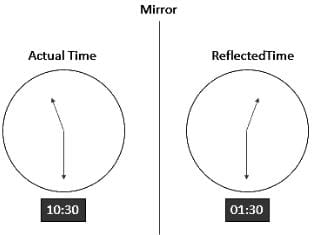Present time is given  by

= 10: 30 + 2 : 15

= 12 : 45

GATE Numerical Reasoning Aptitude (Analytical) Questions - 2 - Question 9

A wire of length 340 mm is to be cut into two parts. One of the parts is to be made into a square and the other into a rectangle where sides are in the ratio of 1:2. What is the length of the side of the square (in mm) such that the combined area of the square and the rectangle is a MINIMUM?

Detailed Solution for GATE Numerical Reasoning Aptitude (Analytical) Questions - 2 - Question 9

Let’s assume that the piece from which rectangle is made, has length x mm.

Perimeter of rectangle = x

∴ Breadth of rectangle = x/6 and length of rectangle = 2x/6 = x/3

⇒ Area of rectangle = x/6 × x/3 = x2/18

Perimeter of square = 340 – x

Length of square = (340 – x)/4 = 85 – x/4

⇒ Area of square =(85 − x/4)2

Total area =(85 − x/4)2 + x2/18 = f(x)

Now, f′(x)=2×(85 − x/4)× − 1 + 2x/18 = 0

Solving, we get: x = 180

Length of square = 85 – x/4 = 85 – 45 = 40 mm

GATE Numerical Reasoning Aptitude (Analytical) Questions - 2 - Question 10

It takes 10s and 15s, respectively, for two trains travelling at different constant speeds to completely pass a telegraph post. The length of the first train is 120 m and that of the second train is 150 m. The magnitude of the difference in the speeds of the two trains (in m/s) is _____.

Detailed Solution for GATE Numerical Reasoning Aptitude (Analytical) Questions - 2 - Question 10

Speed = length / time

⇒ length = speed x time

120 = 10 x s1 ⇒ s= 12

150 = 15 x s⇒ s2 = 10

|s1 - s2| = 2

## General Aptitude for GATE

73 videos|86 docs|108 tests
 Use Code STAYHOME200 and get INR 200 additional OFF Use Coupon Code
Information about GATE Numerical Reasoning Aptitude (Analytical) Questions - 2 Page
In this test you can find the Exam questions for GATE Numerical Reasoning Aptitude (Analytical) Questions - 2 solved & explained in the simplest way possible. Besides giving Questions and answers for GATE Numerical Reasoning Aptitude (Analytical) Questions - 2, EduRev gives you an ample number of Online tests for practice

## General Aptitude for GATE

73 videos|86 docs|108 tests